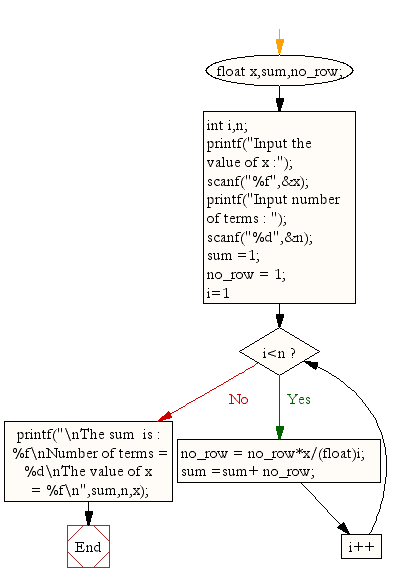﻿ C : Compute the sum of the series [ 1+x+x^2/2!+x^3/3!+...]# C Exercises: Calculate the sum of the series [ 1+x+x^2/2!+x^3/3!+....]

## C For Loop: Exercise-23 with Solution

Write a program in C to display the sum of the series [ 1+x+x^2/2!+x^3/3!+....].

Sample Solution:

C Code:

``````#include <stdio.h>

void main()
{
float x,sum,no_row;
int i,n;
printf("Input the value of x :");
scanf("%f",&x);
printf("Input number of terms : ");
scanf("%d",&n);
sum =1; no_row = 1;
for (i=1;i<n;i++)
{
no_row = no_row*x/(float)i;
sum =sum+ no_row;
}
printf("\nThe sum  is : %f\n",sum);
}
```
```

Sample Output:

```Input the value of x :3
Input number of terms : 5

The sum  is : 16.375000
```

Flowchart:C Programming Code Editor:

Improve this sample solution and post your code through Disqus.

What is the difficulty level of this exercise?

Test your Programming skills with w3resource's quiz.

﻿